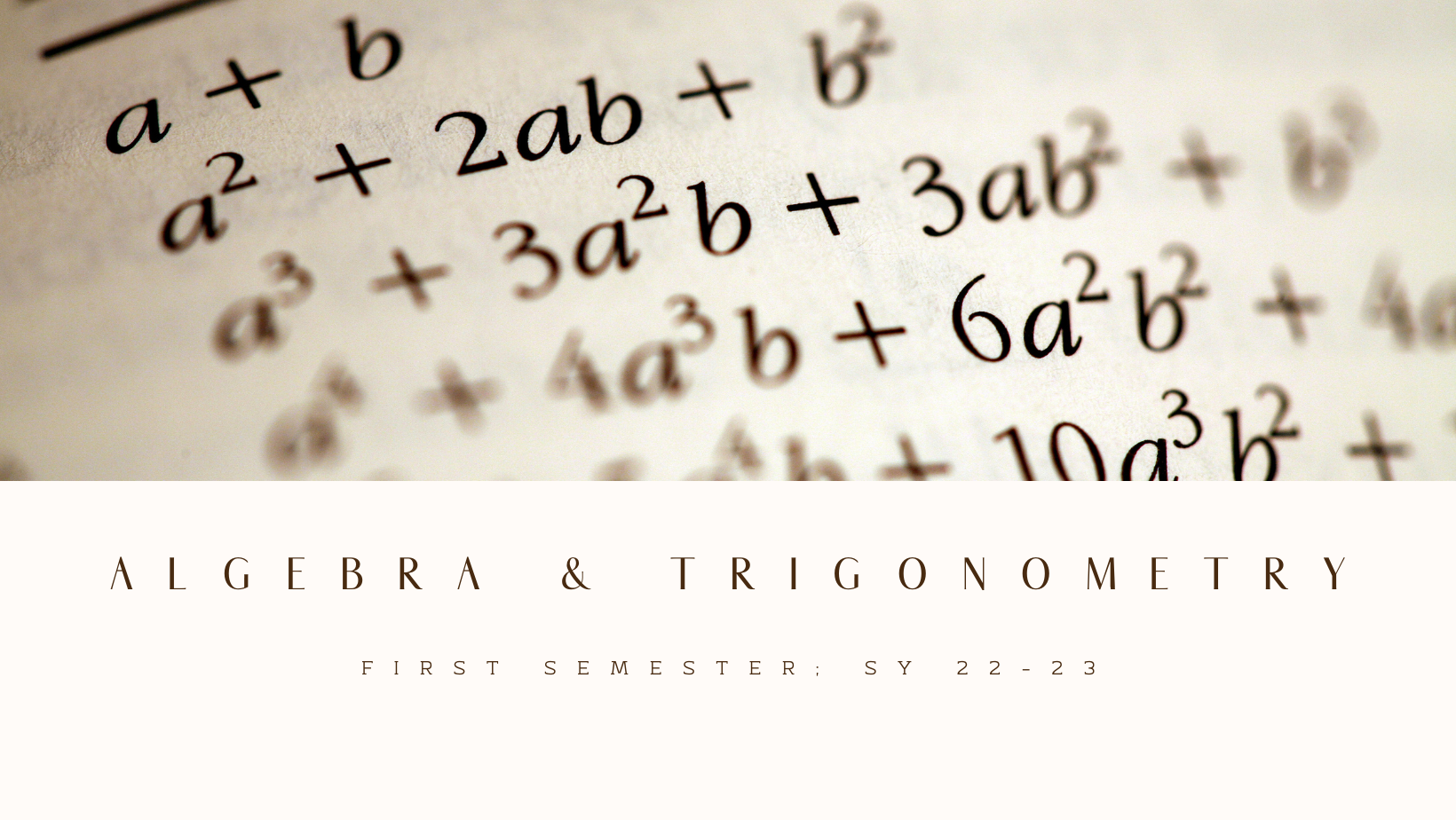#### BA Econ 1B - Algebra and Trigonometry (22-1)

Algebra and Trigonometry is a 3-unit basic core course subject offered to BA Economics students. This subject is designed to prepare students for calculus. The study covers topics such as polynomials, products, and factors; roots, rational exponents; rational expressions; degree and radian angle measure; right triangle; trigonometry and its applications; trigonometric functions and their inverses; and applied problem-solving. The students will be simplifying expressions involving polynomials, radicals, rational, and exponential terms using appropriate properties and rules, use the structure of a function to sketch graphs and verify trigonometric identities by algebraically manipulating expressions using fundamental trigonometric identities

The students are encouraged to devise economic models using the different mathematical tools and techniques discussed based on firms' and consumers' behavior at the end of the course.